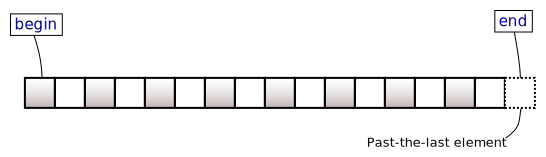/C++

# std::unordered_set<Key,Hash,KeyEqual,Allocator>::begin, std::unordered_set<Key,Hash,KeyEqual,Allocator>::cbegin

 `iterator begin() noexcept;` (1) (since C++11) `const_iterator begin() const noexcept;` (2) (since C++11) `const_iterator cbegin() const noexcept;` (3) (since C++11)

Returns an iterator to the first element of the `unordered_set`.

If the `unordered_set` is empty, the returned iterator will be equal to `end()`.(none).

### Return value

Iterator to the first element.

### Complexity

Constant.

Because both `iterator` and `const_iterator` are constant iterators (and may in fact be the same type), it is not possible to mutate the elements of the container through an iterator returned by any of these member functions.

### Example

```#include <iostream>
#include <unordered_set>

struct Point { double x, y; };

int main()
{
Point pts = {{1, 0}, {2, 0}, {3, 0}};

// points is a set containing the addresses of points
std::unordered_set<Point*> points = { pts, pts + 1, pts + 2 };

// Change each y-coordinate of (i, 0) from 0 into i^2 and print the point
for (auto iter = points.begin(); iter != points.end(); ++iter)
{
(*iter)->y = ((*iter)->x) * ((*iter)->x); // iter is a pointer-to-Point*
std::cout << "(" << (*iter)->x << ", " << (*iter)->y << ") ";
}
std::cout << '\n';

// Now using the range-based for loop, we increase each y-coordinate by 10
for (Point* i : points)
{
i->y += 10;
std::cout << "(" << i->x << ", " << i->y << ") ";
}
}```

Possible output:

```(3, 9) (1, 1) (2, 4)
(3, 19) (1, 11) (2, 14)```